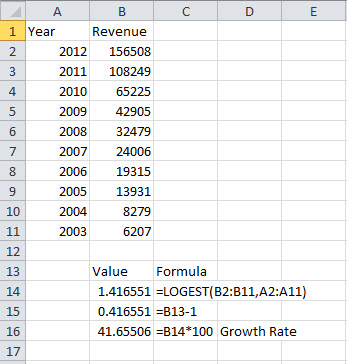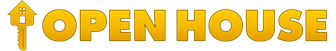# How is the average growth rate calculated?

The average growth rate is calculated by determining the least squares regression line of best fit using the natural log (LN) of the company data. The growth rate is the slope of that line. For data points that are less than or equal to zero, we substitute the very small number of .001 in the calculation.

If you'd like to try this yourself here are a couple of reference websites:

https://science.clemson.edu/physics/labs/tutorials/regression/index.htmlSample Our Resources